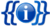# Template:List TOC Letters

 [🔄Purge]Template documentation

## Usage

Similar to {{List TOC}}, this template creates a horizontal table of contents with links from A to Z (controllable) and four optional rows of links. It is intended primarily for list articles where the standard TOC would be unwieldy. The template is styled with the `hlist` class for accessibility and has few superfluous frills.

Unlike {{List TOC}}, the A–Z links do not appear by default. They must be specified as parameters x1 through x26 in order to appear. Additionally, parameters u1 through u4 can be defined to appear before the x* parameters, and z1 through z4 to appear after them.

Two rows to appear above the x* parameters can be defined using a1 through a20 and b1 through b20 and two rows to appear below can be defined using c1 through c20 and d1 through d20.

With the exception of the u* and z* parameters, values are assumed to be anchors on the current page, and are transformed into [[#value|value]] links.

The u* and z* parameter values are rendered as-is, so if they are to be links, you must include proper link markup (i.e. [[value]]).

## Blank parameter list

```{{ListTOCLetters
|a1=|a2=|a3=|a4=|a5=|a6=|a7=|a8=|a9=|a10=|a11=|a12=|a13=|a14=|a15=|a16=|a17=|a18=|a19=|a20=
|b1=|b2=|b3=|b4=|b5=|b6=|b7=|b8=|b9=|b10=|b11=|b12=|b13=|b14=|b15=|b16=|b17=|b18=|b19=|b20=
|u1=|u2=|u3=|u4=
|x1=|x2=|x3=|x4=|x5=|x6=|x7=|x8=|x9=|x10=|x11=|x12=|x13=
|x14=|x15=|x16=|x17=|x18=|x19=|x20=|x21=|x22=|x23=|x24=|x25=|x26=
|z1=|z2=|z3=|z4=
|c1=|c2=|c3=|c4=|c5=|c6=|c7=|c8=|c9=|c10=|c11=|c12=|c13=|c14=|c15=|c16=|c17=|c18=|c19=|c20=
|d1=|d2=|d3=|d4=|d5=|d6=|d7=|d8=|d9=|d10=|d11=|d12=|d13=|d14=|d15=|d16=|d17=|d18=|d19=|d20=
}}```

## Examples

```{{List TOC Letters
|u1=[[List of colors: A–F|A–F]]
|x1=G|x2=H|x3=I|x4=J|x5=K|x6=L|x7=M
|z2=[[List of colors: N–Z|N–Z]]
|d1=Footnotes|d2=References
}}```